# Solving Trigonometric Equations Day 2 Equations with Multiple

• Slides: 9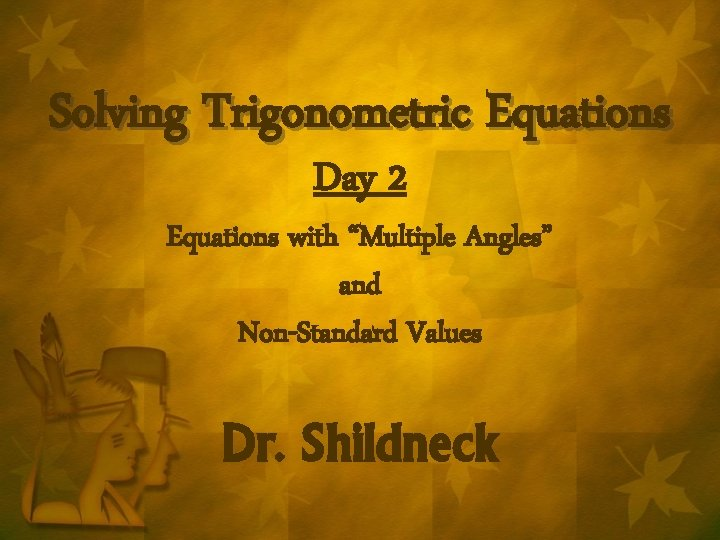Solving Trigonometric Equations Day 2 Equations with “Multiple Angles” and Non-Standard Values Dr. Shildneck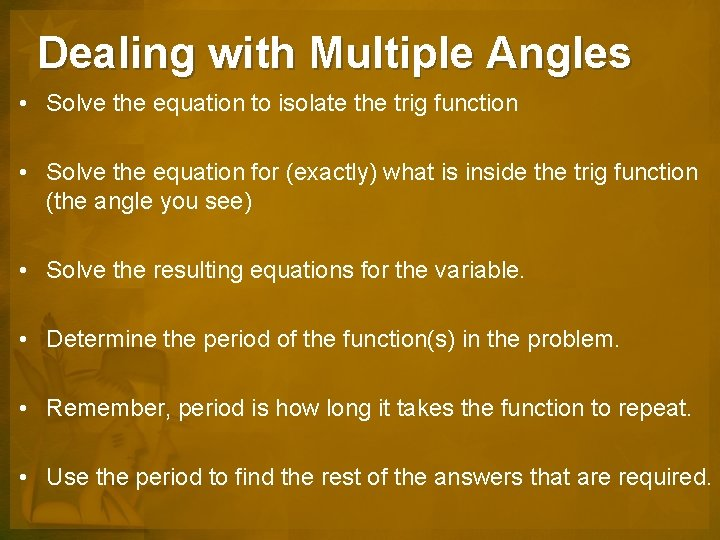Dealing with Multiple Angles • Solve the equation to isolate the trig function • Solve the equation for (exactly) what is inside the trig function (the angle you see) • Solve the resulting equations for the variable. • Determine the period of the function(s) in the problem. • Remember, period is how long it takes the function to repeat. • Use the period to find the rest of the answers that are required.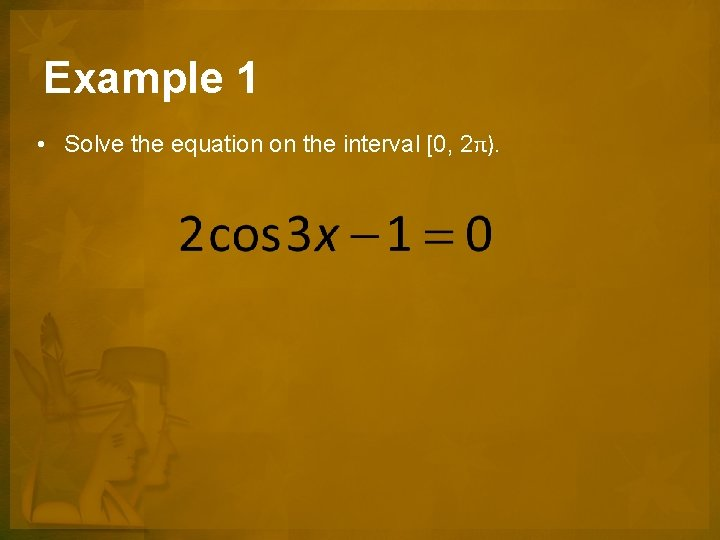Example 1 • Solve the equation on the interval [0, 2π).Example 2 • Solve the equation on the interval [0, 2π).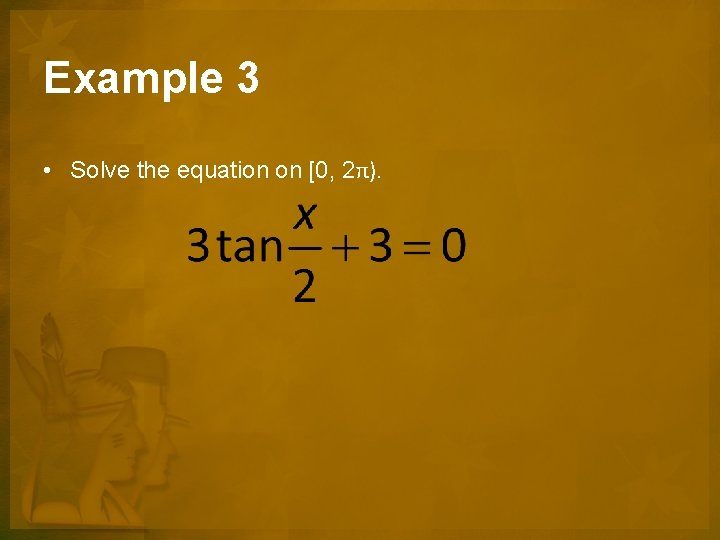Example 3 • Solve the equation on [0, 2π).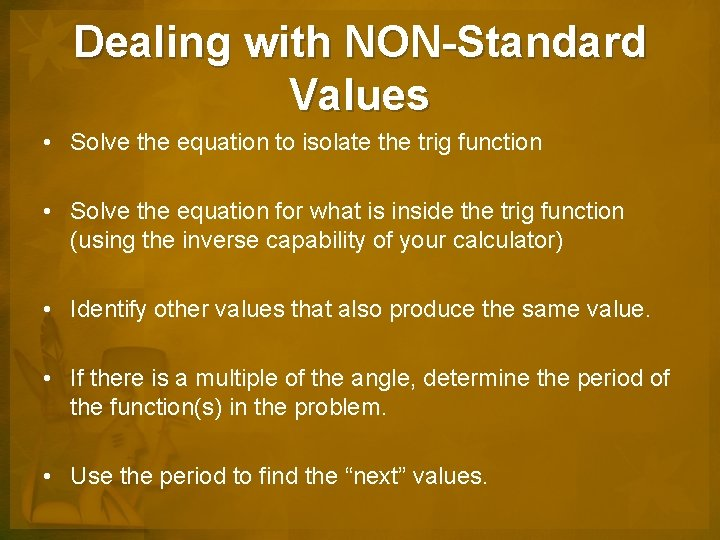Dealing with NON-Standard Values • Solve the equation to isolate the trig function • Solve the equation for what is inside the trig function (using the inverse capability of your calculator) • Identify other values that also produce the same value. • If there is a multiple of the angle, determine the period of the function(s) in the problem. • Use the period to find the “next” values.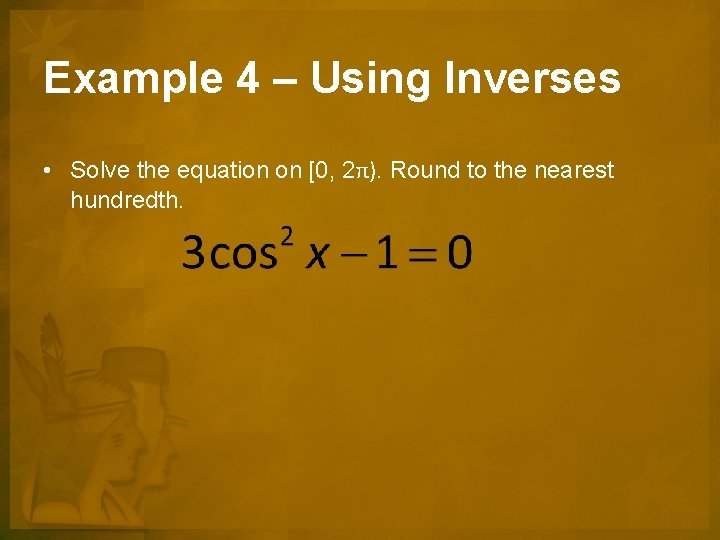Example 4 – Using Inverses • Solve the equation on [0, 2π). Round to the nearest hundredth.Example 5 – Using Inverses • Solve the equation (approximate values).ASSIGNMENT Alt Text P. 376 #57 -87 (odd), 98 -104 Happy Thanksgiving